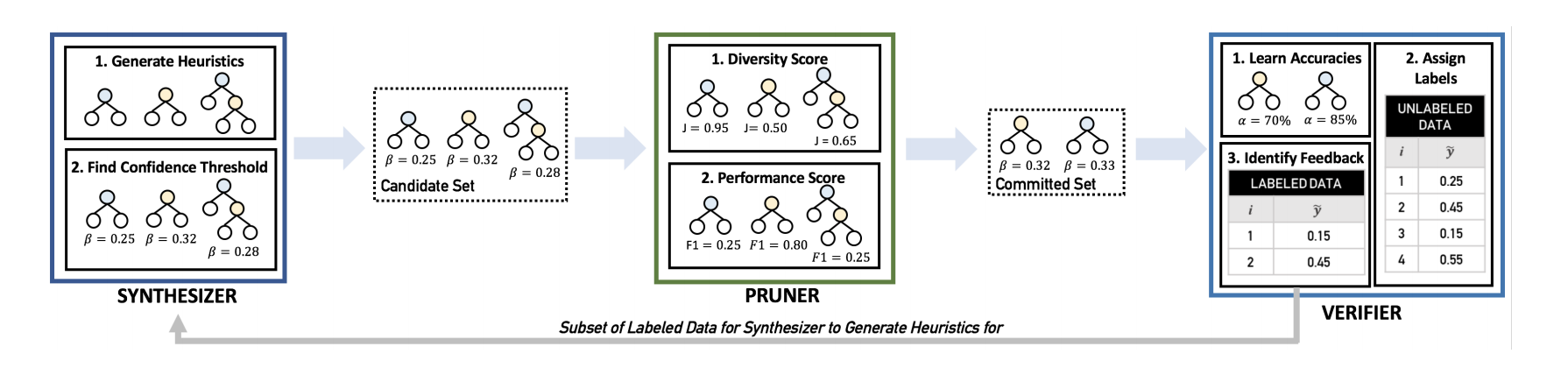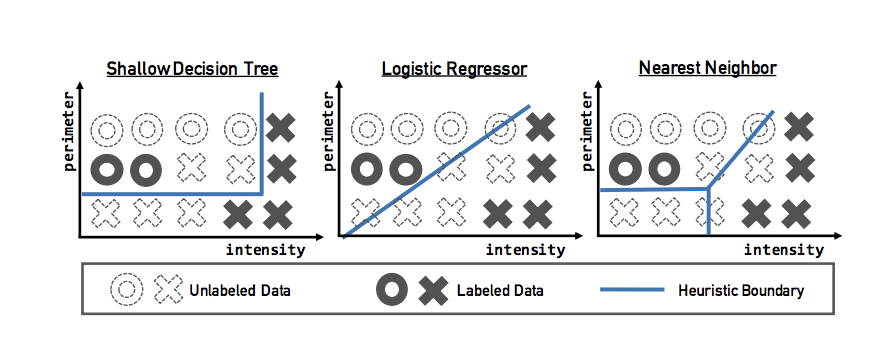Automating Weak Supervision

What is weak supervision?

We recently explored Snorkel, a weak supervision framework for learning when there are limited high-quality labels (see blog post and notebook). To use Snorkel, subject matter experts first write labeling functions to programmatically create labels. Very often these labeling functions attempt to capture heuristics. The labels are then fed into a generative model. The job of the generative model is to estimate the accuracy of the labeling functions while automatically taking into account the pairwise correlation between these functions and labeling propensity (how often a function actually creates a label). Once the generative model is trained, it can be used to estimate the true label for each candidate. The generative model outputs probabilistic labels - numbers between 0 and 1, representing the probability of a positive class. These probabilistic labels can be used to train any end model with a noise-aware loss.

Writing these labeling functions is sometimes not straight-forward; it can be time consuming and expensive. The idea behind Snuba (PDF) is to create a system to “automatically generate heuristics using a small labeled dataset to assign training labels to a large unlabeled dataset.” The labels generated by all these heuristics then feed into a weak supervision framework.

Automatically generating heuristics

Doing this step automatically requires replacing human reasoning that drives heuristic development. The authors take their cue from how humans generate heuristics in order to automate this process. From their observations, subject matter experts often fiddle with the correct threshold for each heuristic in order to make a correct classification. Radiologists, for example, try to figure out a threshold for each heuristic that uses a geometric property of a tumor in order to determine if it is malignant. In addition, subject matter experts tend to develop a single heuristic to assign accurate labels to a subset of the unlabeled data; covering the entire set of unlabeled data requires multiple heuristics. Lastly, humans stop generating heuristics when they have exhausted their domain knowledge.

Inner workings of Snuba

The proposed system works as follows, and requires a small set of labeled data to begin. The labeled data is first transformed into primitives (or features). For tumor images, this might mean numerical features such as area of perimeter of tumor. For text data, this might be one-hot vectors for the bag of words representation. Once we have the primitives, Snuba iteratively generates heuristics on a subset of the input data. Each iteration results in a new heuristic specialized to the subset of data that did not receive high confidence labels from the existing set of heuristics. In addition, the system knows when to stop. All these are accomplished using a three part architecture: synthesizer, pruner, and verifier.Synthesizer

“The synthesizer takes as input the labeled dataset, or a subset of the labeled dataset after the first iteration, and outputs a candidate set of heuristics.” Each heuristic is actually a classification model - a decision stump, a logistic regressor, or a k_nearest neighbor classifier. These models take in primitives (feature representation of the original datapoint) and assign probabilistic labels to the data points. For binary classification, these are probabilities that the input primitive is a 1 (positive label) or a -1 (negative label).Models for creating heuristics (image credit)

These probabilistic labels need to be turned into an actual label (since that’s what a human tries to do with heuristics). A straightforward approach to use probability = 0.5 as a threshold. Any probability less than 0.5 is considered a negative label, any probability above 0.5 is considered a positive label. Snuba builds in a threshold beta around 0.5, so anything greater than 0.5 + beta is a positive label, and anything less than 0.5 - beta is a negative label. All other values result in an “abstained” label. The system tries to find the beta that maximizes the F1 score on the labeled dataset. It does so by iterating through equally spaced values in beta (between 0 and 0.5), calculating the F1 score the heuristic achieves, and selecting beta that maximizes the F1 score. In doing so, Snuba is using the heuristic performance on the small labeled dataset as a proxy for the heuristic performance on the large unlabeled data set.

Pruner

The pruner takes multiple candidate heuristics from the synthesizer and selects one to add to the existing set of heuristics. The goal is to select heuristics that label data points which have never received a label from other heuristics. At the same time, the selected heuristics should perform well when applied to the labeled dataset. To do this, the pruner uses a weighted average of Jaccard distance and F1 score to select the highest ranking heuristic from the candidate set.

Verifier

The verifier takes care of the stopping condition. It uses the label aggregator (the generative model) to produce a single, probabilistic training label for each datapoint in the unlabeled dataset. It also identifies data points in the labeled dataset that receive low confidence labels (probability being close to 0.5). The verifier passes this subset to the synthesizer with the assumption that similar data . points in the unlabeled dataset would have also received low confidence labels. The stopping condition is met “if i) a statistical measure suggests the generative model in the synthesizer is not learning the accuracies of the heuristics properly, or ii) there are no low confidence data points in the small, labeled dataset.” The statistical measure uses the small, labeled dataset to indirectly determine whether the generated heuristics are worse than random for the unlabeled dataset.

Does it work?

The authors show that training labels from Snuba outperform labels from semi-supervised learning and from user-developed heuristics in terms of end model performance for tasks across various domains. These tasks include image classification and text and multi-modal classification.

In some ways Snuba reminds us of active learning - the iterative nature, the need for a stopping condition and the labeled dataset requirement. Active learning relies on the initial small labeled dataset to build a learner (or a model). A selection strategy then picks out data points that are difficult for the model and requests labels for them. The labeled data points (labeled by humans) are added back to the small labeled dataset and the process repeats. The learner gets better as a result. Snuba relies on the initial small labeled dataset to create some heuristics, and continues to use the same small labeled dataset to add more heuristics while evaluating diversity using the unlabeled dataset. Both need a stopping condition and Snuba’s stopping condition is better defined. We think Snuba seems promising, but wonder about the effect of generalizing from a small, labeled dataset to a large, unlabeled dataset.